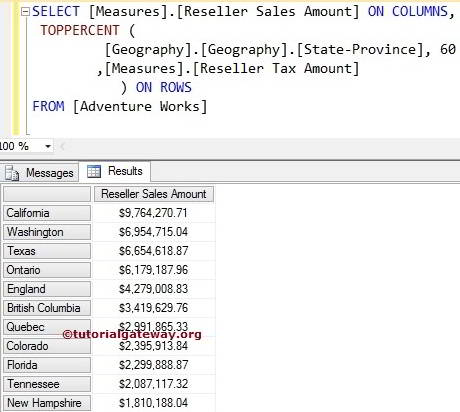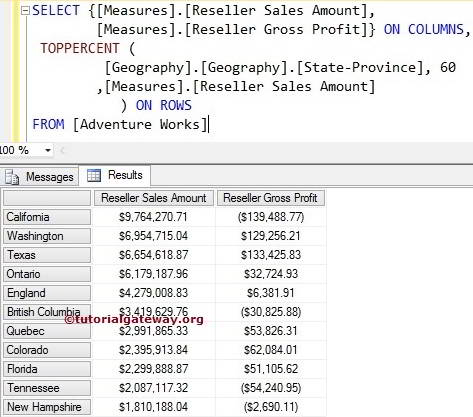# MDX TopPercent Function

The MDX TopPercent function will sort the given data in the descending order. Then selects the required number of records from the sorted data whose total is greater than or equal to the specified Percentage.

## MDX TopPercent Function Syntax

The basic syntax of the MDX TopPercent in Multidimensional Expression is as shown below:

`TOPPERCENT (Set_Expression, Percentage, Numeric_Expression)`
• Set_Expression: Any Multidimensional Expression or attributes on which you want to check.
• Percentage: Please provide the percentage to want to retrieve.
• Numeric_Expression: Any Multidimensional Expression or measure. The MDX TopPercent function will use this Numeric_Expression to sort the data in descending order. And then, picks the number of records required to match the percentage.

Let us see how to write MDX TopPercent function to extract the States from Geography Dimension (present in the Adventure Works Cube) who has contributed 60 percent of total sales in the Reseller Sales amount. For this, we are going to use the below-shown data.

## MDX TopPercent Function Example

The following MDX toppercent query will return all the states present in the Geography Dimension who are contributing 60 Percent of total Reseller sales Amount.

```SELECT {[Measures].[Reseller Sales Amount]} ON COLUMNS,
TOPPERCENT (
[Geography].[Geography].[State-Province], 60
,[Measures].[Reseller Sales Amount]
) ON ROWS

In the above MDX Query, We selected the [Reseller Sales Amount] Measure on the Columns and [State Province] column present in the geography Dimension on Rows. Next, the TopPercent function will sort the data in the descending order using Measures.[Reseller Sales Amount] and then find the percentage of sales from total sales.If you observe the above Toppercent screenshot, they are not the 60 Percent rows of Total records. If you total the Reseller Sales Amounts present in the above screenshot, it will be greater than or equal to 60 percent of the Total Reseller Sales Amount. In short, the states mentioned above are contributing 60 Percent Sales of total sales.

## MDX Toppercent Function Example 2

In this example, We are going to use two measures ([Measures].[Reseller Sales Amount] [Measures].[Reseller Gross Profit]).

```SELECT {[Measures].[Reseller Sales Amount],
[Measures].[Reseller Gross Profit]} ON COLUMNS,
TOPPERCENT (
[Geography].[Geography].[State-Province], 60
,[Measures].[Reseller Sales Amount]
) ON ROWS